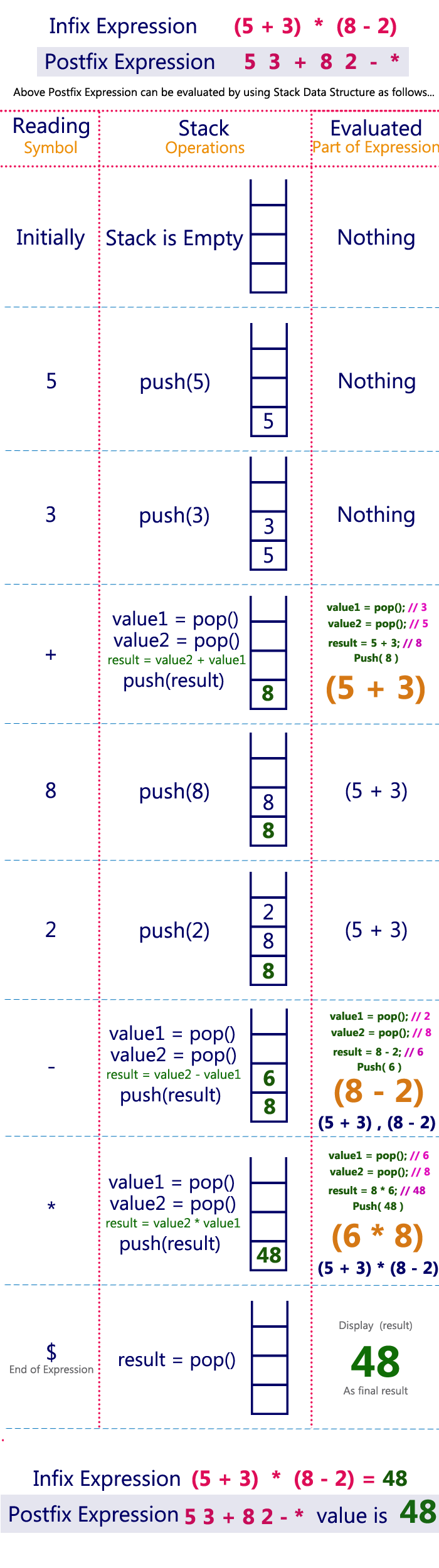The perfect place for easy learning...

×

# Postfix Expression Evaluation

A postfix expression is a collection of operators and operands in which the operator is placed after the operands. That means, in a postfix expression the operator follows the operands.

Postfix Expression has following general structure...

# Example# Postfix Expression Evaluation using Stack Data Structure

A postfix expression can be evaluated using the Stack data structure. To evaluate a postfix expression using Stack data structure we can use the following steps...

1. Read all the symbols one by one from left to right in the given Postfix Expression
2. If the reading symbol is operand, then push it on to the Stack.
3. If the reading symbol is operator (+ , - , * , / etc.,), then perform TWO pop operations and store the two popped oparands in two different variables (operand1 and operand2). Then perform reading symbol operation using operand1 and operand2 and push result back on to the Stack.
4. Finally! perform a pop operation and display the popped value as final result.

# Example

Consider the following Expression...